# Carnot cycleTitle page of a booklet in which Sadi Carnot introduced the cycle and engine named after him. English translation: Reflections on the motive power of fire and on the machines fitted to develop that power by S. Carnot alumnus of the École Polytechnique.

A Carnot cycle is a reversible cycle in the state space of a thermodynamic system. The prototype system going through Carnot cycles is a Carnot engine, a hypothetical engine that converts heat (thermal energy) into work (mechanical energy). A Carnot engine may be thought of to consist of a cylinder with adiabatic lateral walls filled with a substance (the "working medium") that can absorb and deliver heat. The cylinder is closed by an adiabatic piston that can move out and deliver work. The cylinder can receive work by moving the piston inward. A Carnot cycle consists of two isotherms (paths of constant temperature) and two isentropes (paths of constant entropy). A Carnot engine is an idealization of real engines, in actual practice it does not exist.

As any heat engine, a Carnot engine receives an amount of heat Qh from a high-temperature heat reservoir, performs an amount of work W on its surroundings, and delivers remaining heat to a low-temperature heat reservoir. The efficiency of any heat engine is defined as the ratio η ≡ W / Qh, i.e., the ratio of mechanical energy delivered over the thermal energy received. The two most important characteristics of the Carnot heat engine are:

• The efficiency η is independent of the working medium.
• The Carnot cycle is the most efficient reversible cycle possible.

The second characteristic states that other thermodynamic cycles, reversible or irreversible, deliver less work for a given amount of heat, when running between heat reservoirs of the same temperatures as a Carnot engine.

## History

In the early 1820s Sadi Carnot, studying the efficiency of steam engines, conceived a virtual, idealized, version of a steam engine as a vehicle for his Gedankenexperimente (thought experiments) in which he mimicked the work of actual steam engines. He reported the results of his studies in a booklet (see above for its title page) published in 1824. By means of his engine Carnot tried to find answers to questions as: Would it be advantageous to use steam at high pressures, and if so, would there be a limit at which further raising of pressure ceases to be advantageous? How important is the working medium, is steam the best substance, or could any other liquids and their vapors, or air, perform as well?

Conceptually, a steam engine is a very simple device; its rudimentary form consists of a piston that cyclically moves in and out a cylinder, driven by expanding steam. The steam flows from the boiler (hot) to the condenser (cold). Inspired by this construction, Carnot devised an engine, basically a fluid-filled cylinder with a piston, that is alternately exchanging heat with a hot and a cold reservoir. When in contact with the hot reservoir, the engine receives heat and performs work on its surroundings—this stage is comparable to the steam engine piston moving out of the cylinder and performing work. When the cylinder is in contact with the cold reservoir, the surroundings perform work on the engine, i.e., compress the substance inside the cylinder, and the resulting heat is given off to the cold reservoir—this stage is comparable with the piston of a steam engine driven back into the cylinder. While this alternating process is going on, the thermodynamic state of a fixed amount of fluid inside the engine changes periodically, that is, the substance goes through thermodynamic cycles where after each cycle the thermodynamic state is exactly equal to the initial state (has the same temperature, pressure, volume, etc.). Carnot proved that it is irrelevant for his abstract engine what the actually working substance is, it may be water, steam, an ideal gas, or anything else.

At the time of writing his booklet, Carnot saw heat as a fluid, called "caloric", that "falls" from high to low temperature. He thought that the "motive power" that the caloric received from the hot reservoir was converted into work; the caloric itself was conserved and flowed back into the cold reservoir. Carnot was inspired by the equivalence of his engine with a water wheel that, in principle, can extract work from the potential energy ("motive power") of water that is initially stored in a reservoir above the water wheel. Of course, in a water wheel the water itself does not disappear, it is conserved. Caloric may be equated to heat—a form of energy—and motive power (work) is also a form of energy, therefore Carnot's ideas are contrary to conservation of energy—the first law of thermodynamics. Although the first law was not known to Carnot (and it was not even formulated during his life time), Carnot came very close to formulating the second law of thermodynamics, especially in its form given by W. Thomson. For this reason it can be stated that the second law was discovered before the first law.

In the 1850s, R. Clausius and W. Thomson realized that the Carnot engine converts only part of the heat into work. A sizable amount of remaining heat is given off to the cold reservoir and the first law states that the remaining heat plus delivered work are equal to the input heat. Although Carnot's ideas were in contradiction to the first law, nevertheless they proved to be very useful to Clausius and Thomson, when they postulated the second law of thermodynamics.

## Definition

Carnot depicted his engine as an upright cylinder, containing a working medium, with adiabatic walls and its top covered by a movable piston. The base of the cylinder can be changed from adiabatic to heat conducting. The cylinder is placed alternately on top of a cold heat reservoir (of absolute temperature Tc) and on a hot reservoir (of temperature Th). It will be proved below that the nature of the working medium is irrelevant for the thermodynamics of the process, so that it is most convenient to assume that the cylinder is filled with an ideal gas. By the equipartition theorem, the internal energy of one mole of an ideal gas is,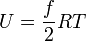$U = \frac{f}{2} RT$

where f, the number of degrees of freedom, is 3 for a monatomic gas, 5 for a diatomic gas, and 6 for a gas of arbitrarily shaped molecules. The quantity R is the molar gas constant. For the present discussion it is important to notice that U is a function of T only.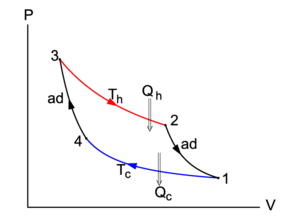Fig. 1. The Carnot cycle in a P-V diagram. The area enclosed by the closed curve is the net work performed by the system (arrows clockwise). The closed curve consists of two adiabatics and two isotherms.

In Figure 1 the Carnot cycle is represented in a P-V diagram, as was first done by Clapeyron in 1834. Consider the compression (decreasing volume) of the gas along the path 1–4. Along this path the cylinder is in thermal equilibrium with the cold reservoir; both have temperature Tc. The environment acts on the piston, moving it slowly (in order to keep the process reversible) into the cylinder; the work done is converted into heat Qc that is given off to the cold reservoir. This process is so slow that the temperature remains constant (i.e., it is an isothermic process), and hence the internal energy U of the gas does not change, dU = 0. By the first and second law of thermodynamics, reference to Figure 1, and the ideal gas law PV = RT (for the sake of argument we consider one mole of gas):$Q_c = T_c \int_1^4 dS = \int_1^4 P dV = RT_c \int_1^4 \frac{dV}{V} \Longrightarrow S_4-S_1 = R \log \frac{V_4}{V_1} < 0,$

where log is the natural (base e) logarithm. The entropy of an ideal gas is a logarithmic function of its volume.

The isotherm 1–4 has the following functional form in the P-V plane,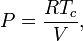$P = \frac{RT_c}{V},$

clearly P is a function of V.

At point 4 the cylinder is removed from the cold reservoir, its base is changed to adiabatic, so that the whole cylinder, including the piston, cannot exchange heat with the surroundings. The piston is moved inward from V4 to V3 and by this compression the gas is heated up to exactly the temperature Th of the hot reservoir, for in order that heat can be exchanged reversibly between the cylinder and the hot reservoir it is necessary that cylinder and reservoir have the same temperature. From 4 to 3 the path in the P-V diagram is adiabatic and reversible. The entropy does not change during this compression, dS = 0 (an isentropic process). It is easy to derive the equation for the adiabatic in the P-V plane,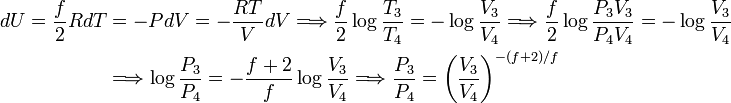\begin{align} dU = \frac{f}{2} R dT &= -PdV = - \frac{RT}{V} dV \Longrightarrow \frac{f}{2} \log\frac{T_3}{T_4} = -\log\frac{V_3}{V_4} \Longrightarrow \frac{f}{2} \log\frac{P_3 V_3}{P_4 V_4} = -\log\frac{V_3}{V_4}\\ &\Longrightarrow \log\frac{P_3}{P_4} = -\frac{f+2}{f} \log\frac{V_3}{V_4} \Longrightarrow \frac{P_3}{P_4} = \left(\frac{V_3}{V_4}\right)^{-(f+2)/f} \end{align}

For a monatomic gas (f=3) the functional form of the curve 4-3 is:$P = c\; V^{-5/3}\quad\hbox{with}\quad c\equiv P_4\,V_4^{5/3}.$

The integral$\int_1^3 PdV$ is the work done by the surroundings on the cylinder. In the P-V diagram it is the negative of the surface bounded by the curve 1–4–3 and the V axis.

At point 3 the base of the cylinder, with temperature Th, is changed back to heat-conducting, and the cylinder is placed on top of the hot reservoir. Slowly the piston moves out, meaning that the cylinder performs work on the surroundings. While this happens the cylinder receives in total the heat Qh from the hot reservoir. The isotherm 3–2 has the functional form P(V) = RTh/V and the entropy increases:$S_2-S_3 = R \log\frac{V_2}{V_3} > 0.$

From 2 to 1 isentropic expansion occurs, work is done by the cylinder on the surroundings, and its temperature decreases to the temperature Tc of the cold reservoir. The integral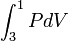$\int_3^1 PdV$ is the work done by the cylinder. In the P-V diagram it is the surface bounded by the curve 3–2–1 and the V axis. Note that this surface is larger than the surface below the curve 1–4–3. In total the work done during the cycle is difference of the the two surfaces:

The total work done by the Carnot engine is the area enclosed by the closed path in the PV-diagram.

By the first law of thermodynamics, the total work W is equal to QhQc, part of the heat Qh received from the hot reservoir is converted into work, part is passed on to the cold reservoir.

The process, as depicted in Figure 1, runs clockwise. All the processes are reversible, so that all arrows can be reverted. The integral over the upper path from 1, 2 to 3 is then the negative of the area under this curve and represents the work done by the surroundings on the system (cylinder). The integral from 3, 4 to 1 gives the work done by the system. In short, when the arrows run anti-clockwise, the area enclosed by the closed curve in Figure 1 is the work done by the surroundings on the system. This work results in a extraction of heat Qc = QhW from the cold reservoir, i.e., the engine works as a heat pump when it runs in anti-clockwise direction.

## Comparison with other thermodynamic cycles

A Carnot cycle can be represented in a T-S diagram, with T along the y-axis and S (entropy) along the x-axis. The isotherms are parallel horizontal lines at Tc and Th. The isentropes are parallel vertical lines (by definition they are of constant entropy). Thus, a Carnot cycle is represented by a rectangle. The surface of the rectangle is the net heat absorbed (arrows clockwise), or the net heat released (arrows counter-clockwise) by the Carnot cycle. By the first law, these areas are equal to the work done by the cycle, or on the cycle, respectively.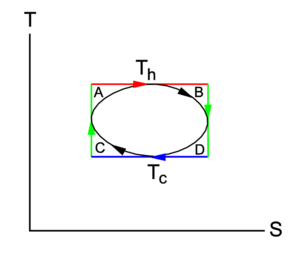Fig. 2. T-S diagram of an arbitrary thermodynamic cycle (black). A Carnot cycle (the rectangle with red and blue isotherm and two green isentropes) circumscribes the cycle. A, B, C, and D indicate the areas of the corresponding patches.

Consider an arbitrary cycle that is situated between Tc and Th in a T-S diagram, see the closed black curve in Figure 2. Apart from its temperature limits, the cycle is arbitrary, it may even be irreversible. Any arbitrary cycle can be circumscribed by a Carnot cycle, as is shown in Figure 2. It will now be shown that the efficiency η of the circumscribing Carnot cycle is larger than that of the inscribed cycle. Since the second law states that the efficiency of an irreversible cycle is always less than that of the corresponding reversible cycle, it can be assumed in this proof that the arbitrary cycle is reversible, irreversibility can only decrease its efficiency. The quantities pertaining to the arbitrary cycle are primed, those belonging to the Carnot cycle are unprimed.

Remembering that Qh is the area bounded by the red (Th) isotherm and the S-axis and that Qc is the area bounded by the blue (Tc) isotherm and the S-axis, one sees that the heat absorbed and released by the arbitrary cycle is connected to the heat absorbed and released by the Carnot cycle through the following expression for the respective areas shown in Figure 2,$Q'_h = Q_h - A - B \quad\hbox{and}\quad Q'_c = Q_c + C + D.$

The efficiency η′ of the arbitrary cycle is given by,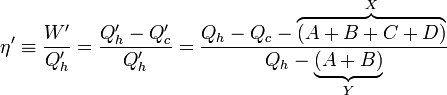$\eta' \equiv \frac{W'}{Q'_h}= \frac{Q'_h-Q'_c}{Q'_h}= \frac{Q_h-Q_c -\overbrace{(A+B+C+D)}^{X}}{Q_h-\underbrace{(A+B)}_{Y}}$

Now η′ <η, because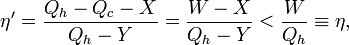$\eta' = \frac{Q_h-Q_c -X}{Q_h-Y} =\frac{W -X}{Q_h-Y} < \frac{W}{Q_h} \equiv \eta,$

as follows thus,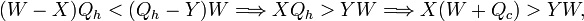$(W -X)Q_h < (Q_h-Y) W \Longrightarrow X Q_h > Y W \Longrightarrow X(W+Q_c) > YW,$

and because X > Y and X and Qc are positive, the inequality η′ <η follows. Conclusion:

The Carnot cycle is the most efficient thermodynamic cycle for given temperature limits.

The proof given here is taken from Ref.

### Remark on the efficiency of reversible cycles

It is very frequently stated that the efficiency of all reversible cycles, operating between the same temperatures, is the same as the efficiency of the corresponding Carnot cycle, see for instance. This is in contradiction to what is just proved. In most texts it is unspecified what the exact meaning is of "operating between the same temperatures". Along the arbitrary (black) cycle in Figure 2, one finds varying temperatures, only the maximum and minimum temperatures are the same as those of the Carnot cycle. Because the belief that all reversible cycles operate with maximum (i.e., Carnot) efficiency is widespread, it is useful to look into it in more detail.

The first question, that must be answered, is how in a Gedankenexperiment an arbitrary (non-Carnot) cycle could be implemented, for it is difficult to see how a heat reservoir of fixed temperature Th can transfer heat reversibly to an engine with a working medium of temperature less than Th. Indeed, as soon as there is a temperature gradient, heat will flow spontaneously, i.e., irreversibly. It is exactly for this reason that a Carnot cycle absorbs and releases heat along isotherms that have their temperatures exactly equal to those of the reservoirs with which the cycle exchanges heat.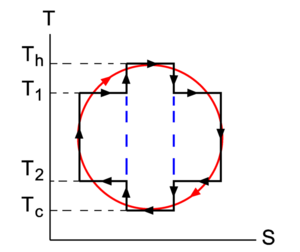Fig. 3. An arbitrary cycle (red) in the T-S diagram is approximated by three Carnot cycles (rectangles). The internal (blue dashed) isentropes are passed twice, once up and once down, all state changes along these internal lines are canceled.

An implementation of a non-Carnot cycle could be achieved by having a (large) number of heat reservoirs with different temperatures and by approximating the arbitrary cycle by a (large) number of Carnot cycles operating between pairs of these reservoirs. In Figure 3 an example is sketched of an arbitrary cycle (red) that is approximated by three Carnot cycles (the black + dashed blue rectangles) that operate from left to right between T1 > T2, Th > Tc, and again between T1 > T2. Four heat reservoirs are needed in this example, two hot and two cold. Recalling that an area in the T-S diagram is equal to an amount of heat, one finds that efficiency of the left- and right-most rectangles is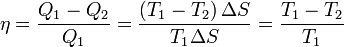$\eta = \frac{Q_1 - Q_2}{Q_1} = \frac{(T_1 -T_2) \,\Delta S}{ T_1 \Delta S} = \frac{T_1-T_2}{T_1}$

which is evidently less than the efficiency of the Carnot cycle in the middle,$\eta_\mathrm{max} = \frac{T_h-T_c}{T_h}.$

The latter efficiency is equal to the efficiency of the single Carnot cycle that circumscribes the whole red cycle. The overall efficiency of the three Carnot cycles that approximate the red cycle is less than that of the single Carnot cycle circumscribing the arbitrary (red) cycle.

Clearly, an arbitrary reversible thermodynamic cycle can be implemented by the aid of a (large) number of heat reservoirs, all with temperatures bracketed by Th and Tc. Between pairs of heat reservoirs (with T1 > T2) Carnot cycles can operate, all with an efficiency less than the maximum efficiency ηmax. (Note that T2 > Tc and T1 < Th). The overall efficiency of the process, approximated by many Carnot cycles, is less than the efficiency of the single Carnot cycle that circumscribes the arbitrary cycle.

## Different Carnot cycles

The following result is an almost direct consequence of Thomson's formulation of the second law:

Two arbitrary and different Carnot cycles with the same hot and cold temperature have the same efficiency, regardless of their working media.

To support the proof, Figure 4 shows two different Carnot engines (green circles) with different working media coupled to the same hot and cold heat reservoir. Because both Carnot cycles describe a rectangle in the T-S diagram (see previous section), it can be easily arranged that the input heat to the two engines is exactly the same, namely Qh. In general the internal energies of the two working media change differently under the influx of heat. That is, although (in the definition of the vertex numbers in Figure 1),$Q_h= \int_2^3 dU^\mathrm{a} + \int_2^3 P^\mathrm{a} dV^\mathrm{a} = \int_2^3 dU^\mathrm{b} + \int_2^3 P^\mathrm{b} dV^\mathrm{b},$

in general$\int_2^3 dU^\mathrm{a} \ne \int_2^3 dU^\mathrm{b}.$

However, after a full cycle this difference is of no concern, because the internal energy is a state variable, i.e, returns to the same value upon a full cycle. In other words, the internal energy gained or lost in half a cycle is lost or gained in the other half.

Suppose the leftmost engine in Figure 4 delivers more work than the rightmost, Wa > Wb. By the first law this implies that Qac < Qbc. Revert the second (right) engine, then the two amounts of heat Qh leaving and entering the hot reservoir are the same. This means that the hot reservoir may be removed and heat leaving the right engine can be piped directly into the left engine. The two engines together perform work WaWb > 0 on their surroundings, and the second reservoir cools down, QacQbc < 0. However, this is in violation of Thomson's principle (no work can be obtained by cooling down a single heat source) and it must be concluded that the assumption Wa > Wb leads to a contradiction.

Next assume that Wa < Wb. By the very same reasoning it follows that this also leads to a violation of the second law, and hence it must be concluded that Wa = Wb. By assumption the input heat to the two engines is the same, so that$\eta^\mathrm{a} \equiv \frac{W^\mathrm{a} }{Q_h} = \eta^\mathrm{b} \equiv \frac{W^\mathrm{b} }{Q_h}.$